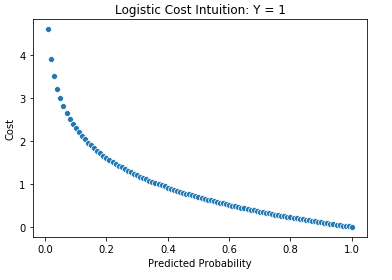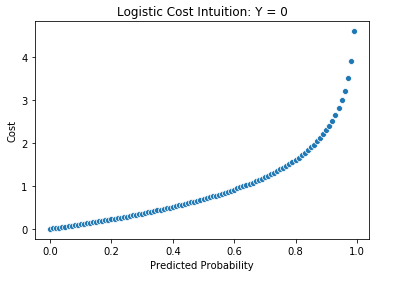# What is the error / loss function in logistic regression?

Logistic regression uses a logistic loss function, where the cost for a single observation is represented by:

and the overall loss function is a sum of the individual costs assigned to each observation.

Using this loss function ensures that a higher penalty is given to observations with a predicted probability close to 0 when it actually belongs to the positive class, and likewise for observations with a predicted probability close to 1 that actually belong to the negative class. This can be conceptualized by considering separate cases when y is both 1 and 0. In the former case, the second term of the cost function goes away, and the cost associated with each probability is shown in the plot below. It can be seen that the cost decreases when the predicted probability is closer to 1 and increases as it approaches 0, which makes sense when the actual label is 1.On the other hand, if Y=0, the first term of the cost function goes away, and the relationship between the predicted probability and the remaining term is shown below. Inversely to the case when Y=1, the cost decreases as the prediction approaches 0 and increases as it approaches 1, as one would expect.Find out all the ways
that you can